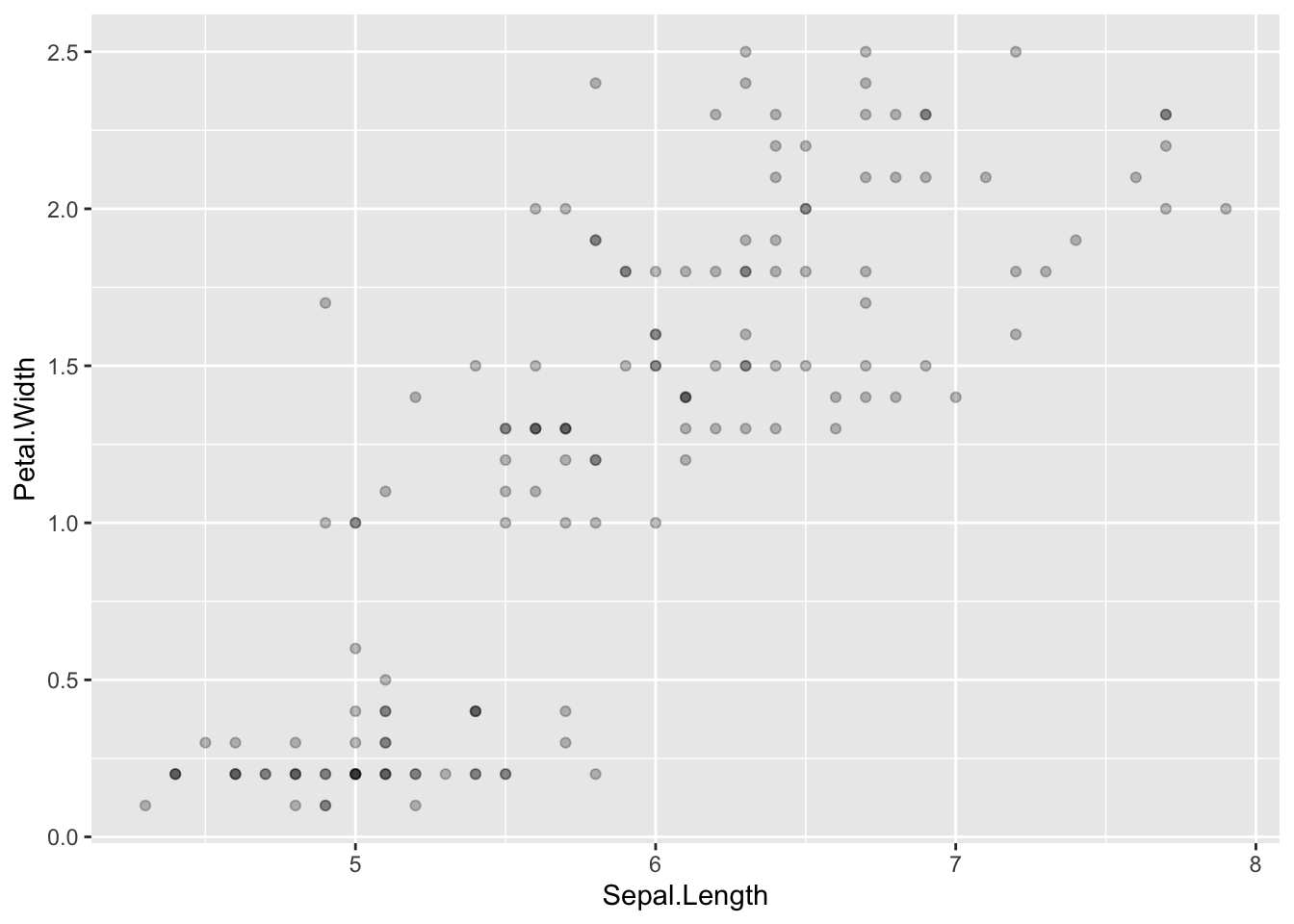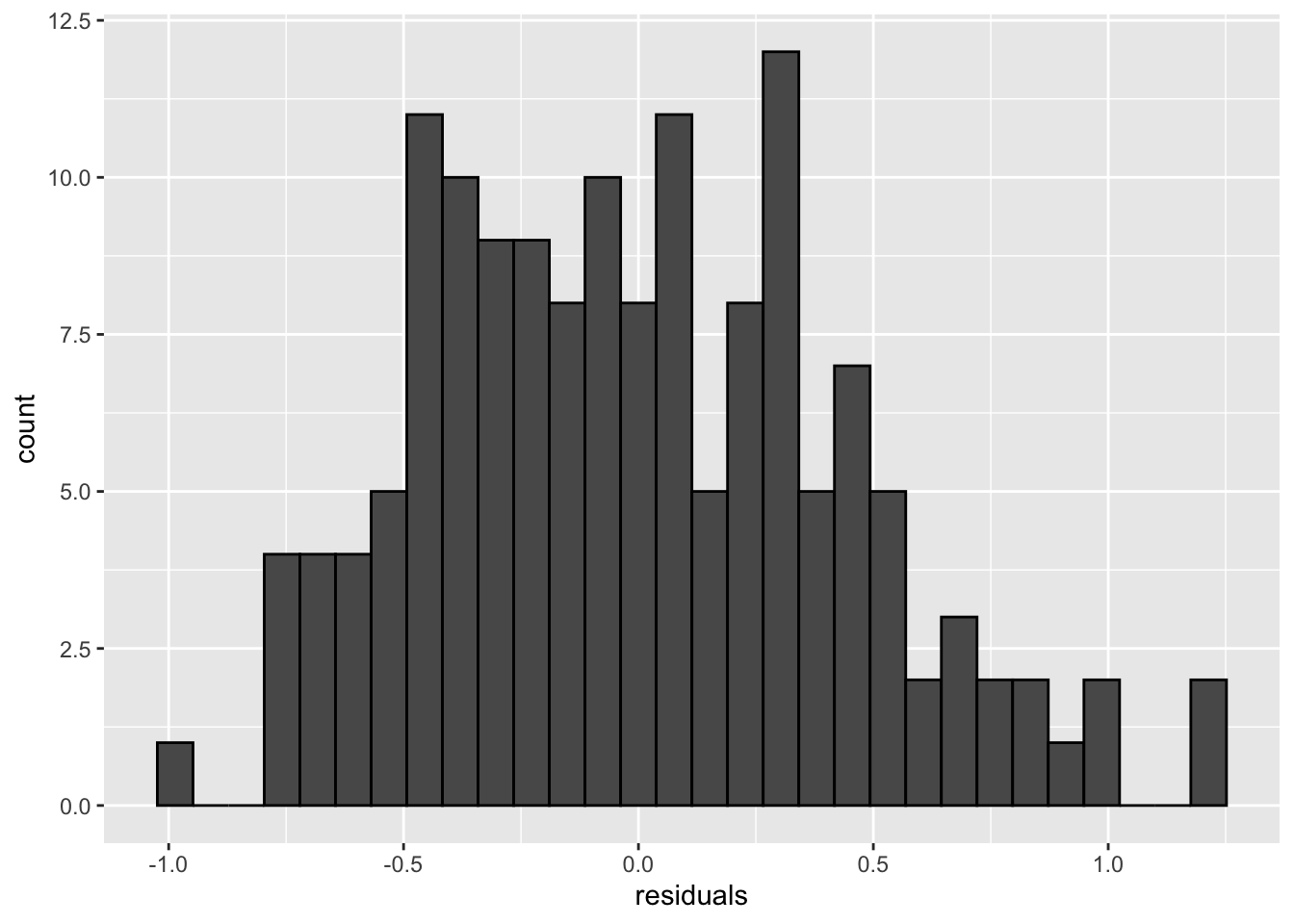## The iris dataset

There is a built-in dataset called iris that has measurements of different parts of flowers. (See ?iris for information about the dataset.)

### Question 1

Use ggplot2 to make a scatterplot that visualizes the relationship between sepal length (horizontal axis) and petal width (vertical axis). Watch out for overplotting.

ggplot(iris, aes(Sepal.Length, Petal.Width)) +
geom_point(alpha = .25)### Question 2

Run a regression model that predicts the petal width from the sepal length, and store the model object in the variable iris_mod. End the block by printing out the summary of the model.

iris_mod <- lm(Petal.Width ~ Sepal.Length, iris)

summary(iris_mod) #print out the model summary
##
## Call:
## lm(formula = Petal.Width ~ Sepal.Length, data = iris)
##
## Residuals:
##      Min       1Q   Median       3Q      Max
## -0.96671 -0.35936 -0.01787  0.28388  1.23329
##
## Coefficients:
##              Estimate Std. Error t value Pr(>|t|)
## (Intercept)  -3.20022    0.25689  -12.46   <2e-16 ***
## Sepal.Length  0.75292    0.04353   17.30   <2e-16 ***
## ---
## Signif. codes:  0 '***' 0.001 '**' 0.01 '*' 0.05 '.' 0.1 ' ' 1
##
## Residual standard error: 0.44 on 148 degrees of freedom
## Multiple R-squared:  0.669,  Adjusted R-squared:  0.6668
## F-statistic: 299.2 on 1 and 148 DF,  p-value: < 2.2e-16

### Question 3

Make a histogram of the residuals of the model using ggplot2.

residuals <- residuals(iris_mod)

ggplot() +
geom_histogram(aes(residuals), color = "black")### Question 4

Write code to predict the petal width for two plants, the first with a sepal length of 5.25cm, and the second with a sepal length of 6.3cm. Store the vector of predictions in the variable iris_pred.

iris_pred <- predict(iris_mod, tibble(Sepal.Length = c(5.25, 6.3)))

iris_pred # print the predicted values
##         1         2
## 0.7526022 1.5431657

## Simulating data from the linear model

### Question 5

NOTE: You can knit this file to html to see formatted versions of the equations below (which are enclosed in $ characters); alternatively, if you find it easier, you can hover your mouse pointer over the $ in the code equations to see the formatted versions.

Write code to randomly generate 10 Y values from a simple linear regression model with an intercept of 3 and a slope of -7. Recall the form of the linear model:

$$Y_i = \beta_0 + \beta_1 X_i + e_i$$

The residuals ($$e_i$$s) are drawn from a normal distribution with mean 0 and variance $$\sigma^2 = 4$$, and $$X$$ is the vector of integer values from 1 to 10. Store the 10 observations in the variable Yi below. (NOTE: the standard deviation is the square root of the variance, i.e. $$\sigma$$; rnorm() takes the standard deviation, not the variance, as its third argument).

X <- 1:10
err <- rnorm(10, sd = 2)
Yi <- 3 - 7 * X + err

Yi # print the values of Yi
##    -3.535761  -9.007200 -17.938710 -25.647394 -35.984147 -36.451994
##   -42.896233 -54.699763 -62.737743 -64.578007

### Question 6

Write a function to simulate data with the form.

$$Y_i = \beta_0 + \beta_1 X_i + e_i$$

The function should take arguments for the number of observations to return (n), the intercept (b0), the effect (b1), the mean and SD of the predictor variable X (X_mu and X_sd), and the SD of the residual error (err_sd). The function should return a tibble with n rows and the columns id, X and Y.

sim_lm_data <- function(n = 100, b0 = 0, b1 = 0,
X_mu = 0, X_sd = 1, err_sd = 1) {
tibble(
id = 1:n,
X = rnorm(n, X_mu, X_sd),
err = rnorm(n, 0, err_sd),
Y = b0 + b1*X + err
) %>%
select(id, X, Y)
}

dat6 <- sim_lm_data(n = 10) # do not edit

knitr::kable(dat6) # print table
id X Y
1 -1.1323655 1.3350575
2 -0.7875753 -1.2133955
3 0.8764906 -0.6973375
4 1.0195403 -0.3126511
5 -0.2536588 1.2321988
6 -0.3274184 0.7764865
7 -0.5838995 0.5046358
8 0.6254302 0.8408165
9 -0.4307918 -2.0545288
10 -0.1372466 1.8822383

### Question 7

Use the function from Question 6 to generate a data table with 10000 subjects, an intercept of 80, an effect of X of 0.5, where X has a mean of 0 and SD of 1, and residual error SD of 2.

Analyse the data with lm(). Find where the analysis summary estimates the values of b0 and b1. What happens if you change the simulation values?

dat7 <- sim_lm_data(n = 10000, b0 = 80, b1 = 0.5,
X_mu = 0, X_sd = 1, err_sd = 2)

mod7 <- lm(Y ~ X, data = dat7)

summary(mod7) # print summary
##
## Call:
## lm(formula = Y ~ X, data = dat7)
##
## Residuals:
##     Min      1Q  Median      3Q     Max
## -7.3088 -1.3201 -0.0242  1.3288  7.0015
##
## Coefficients:
##             Estimate Std. Error t value Pr(>|t|)
## (Intercept) 80.04364    0.01964 4075.33   <2e-16 ***
## X            0.50430    0.01983   25.43   <2e-16 ***
## ---
## Signif. codes:  0 '***' 0.001 '**' 0.01 '*' 0.05 '.' 0.1 ' ' 1
##
## Residual standard error: 1.964 on 9998 degrees of freedom
## Multiple R-squared:  0.06074,    Adjusted R-squared:  0.06065
## F-statistic: 646.6 on 1 and 9998 DF,  p-value: < 2.2e-16

### Question 8

Use the function from Question 6 to calculate power by simulation for the effect of X on Y in a design with 50 subjects, an intercept of 80, an effect of X of 0.5, where X has a mean of 0 and SD of 1, residual error SD of 2, and alpha of 0.05.

Hint: use broom::tidy() to get the p-value for the effect of X.

# ... lets you include any arguments to send to sim_lm_data()
sim_lm_power <- function(...) {
dat <- sim_lm_data(...)
lm(Y~X, dat) %>%
broom::tidy() %>%
filter(term == "X") %>%
pull(p.value)
}

p_values <- replicate(1000, sim_lm_power(n = 50, b0 = 80, b1 = 0.5, X_mu = 0, X_sd = 1, err_sd = 2))

power <- mean(p_values < .05)

power # print the value
##  0.4

### Question 9

Calculate power (i.e., the false positive rate) for the effect of X on Y in a design with 50 subjects where there is no effect and alpha is 0.05.

p_values <- replicate(1000, sim_lm_power(n = 50))

false_pos <- mean(p_values < .05)

false_pos # print the value
##  0.045